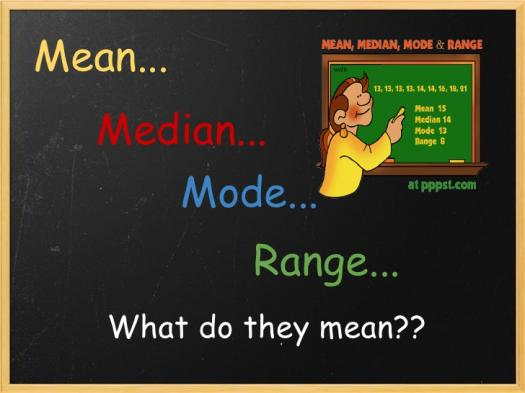# Mean Median Mode And Range

8 Questions | Total Attempts: 106Mean, median, and the mode is three kinds of "averages". There are many "averages" in statistics, but these are, I think, the three most common, and are certainly the three you are most likely to encounter in your pre-statistics courses if the topic comes up at all.

• 1.
What is the mean of these numbers ? 10, 5, 3, 2, 6, 7
• A.

5.2

• B.

5.5

• C.

5

• D.

5.7

• 2.
Find the mode of these numbers 3 5 8 6 4 3 4 3
• A.

8

• B.

4

• C.

3

• D.

6

• 3.
Which number is the median ? 5 7 8 2 1
• A.

5

• B.

7

• C.

8

• D.

2

• 4.
When calculated the mean was 6. Complete the missing number in order to calculate the mean. 4,6,10,2, ?
• A.

9

• B.

5

• C.

1

• D.

8

• 5.
Jack was trying to work out the mode. Which of the following statements will help jack do so?
• A.

The highest number subtract the lowest number ?

• B.

Add the numbers up and divide by the amount of numbers

• C.

The most common number

• D.

Place the numbers in order and find the middle number

• 6.
Can you find the mean.? 4,8,12,2,24,10
• A.

60

• B.

58

• C.

59

• D.

48

• 7.
Which is the correct meaning of the word median?
• A.

The numbers are placed in order and added up

• B.

The numbers are placed in order and the middle number is the median

• C.

The median is the number with the biggest value

• D.

The median is the smallest number

• 8.
Susan adds up 6 numbers then divides them by six. Which method of calculation is she using?
• A.

Median

• B.

Mean

• C.

Mode

• D.

Range

Related TopicsBack to top